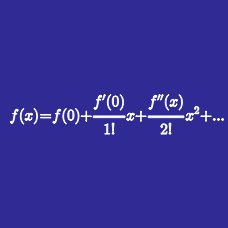Calculus

# Taylor Series Approximation

Let $$f(x)$$ be a function such that $$f(0)=3, f'(0)=1,$$ and $$f(3)=1.$$ Using a quadratic Taylor polynomial of $$f(x),$$ we can approximate the value of $$f''(0)$$ as $f''(0) \approx -\frac{A}{B},$ where $$A$$ and $$B$$ are coprime integers. Find the value of $$AB.$$

Using the quadratic Taylor polynomial of $$f(x) = \frac{1}{x}$$ at $$x=5,$$ find the approximate value of $$\frac{1}{7}$$ multiplied by $$5^3.$$

For the linear Taylor polynomial $$g(x)=ax+b$$ of $$f(x)=-\frac{1}{x-1}$$ at $$x=2,$$ find the error $$e$$ defined by $e=\int_{2}^{3}{(g(x)-f(x))^2 dx}.$

Using the quadratic Taylor polynomial of $$f(x) = \ln{x}$$ at $$x=4,$$ we can approximate the value of $$\ln{9}$$ in the form $\ln{A}+B,$ where $$A$$ and $$B$$ are rational numbers. Find the value of $$2A^2B.$$

Let $$f(x)$$ be a function such that $$f(0)=1, f'(0)=2,$$ and $$f(3)=1.$$ Using a quadratic Taylor polynomial of $$f(x),$$ we can approximate the value of $$f''(0)$$ as $f''(0) \approx -\frac{A}{B},$ where $$A$$ and $$B$$ are coprime integers. Find the value of $$AB.$$

×